# JP4988883B2 - Comparator circuit - Google Patents

## Info

Publication number
JP4988883B2
JP4988883B2 JP2010044369A JP2010044369A JP4988883B2 JP 4988883 B2 JP4988883 B2 JP 4988883B2 JP 2010044369 A JP2010044369 A JP 2010044369A JP 2010044369 A JP2010044369 A JP 2010044369A JP 4988883 B2 JP4988883 B2 JP 4988883B2
Authority
JP
Japan
Prior art keywords
current
bias current
circuit
differential amplifier
comparator circuit
Prior art date
Legal status (The legal status is an assumption and is not a legal conclusion. Google has not performed a legal analysis and makes no representation as to the accuracy of the status listed.)
Active
Application number
JP2010044369A
Other languages
Japanese (ja)
Other versions
JP2011182188A (en
Inventor

Original Assignee

Priority date (The priority date is an assumption and is not a legal conclusion. Google has not performed a legal analysis and makes no representation as to the accuracy of the date listed.)
Filing date
Publication date
Application filed by 株式会社半導体理工学研究センター filed Critical 株式会社半導体理工学研究センター
Priority to JP2010044369A priority Critical patent/JP4988883B2/en
Publication of JP2011182188A publication Critical patent/JP2011182188A/en
Application granted granted Critical
Publication of JP4988883B2 publication Critical patent/JP4988883B2/en
Active legal-status Critical Current
Anticipated expiration legal-status Critical

• 230000003044 adaptive Effects 0 claims description 118
• 230000000694 effects Effects 0 description 7
• 238000005516 engineering processes Methods 0 description 4
• 238000000034 methods Methods 0 description 4

## Images

•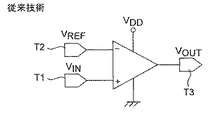•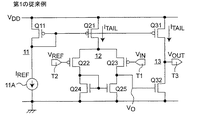•••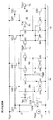•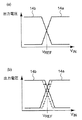•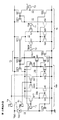••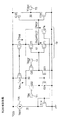•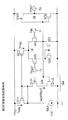•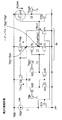•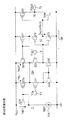•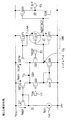•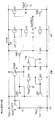••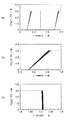••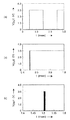•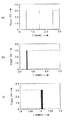•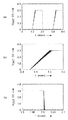•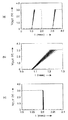•••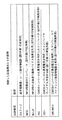•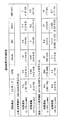••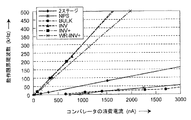••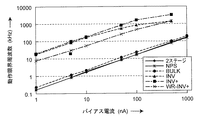•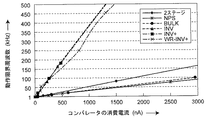••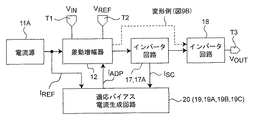## Classifications

• HELECTRICITY
• H03BASIC ELECTRONIC CIRCUITRY
• H03KPULSE TECHNIQUE
• H03K5/00Manipulating of pulses not covered by one of the other main groups of this subclass
• H03K5/22Circuits having more than one input and one output for comparing pulses or pulse trains with each other according to input signal characteristics, e.g. slope, integral
• H03K5/24Circuits having more than one input and one output for comparing pulses or pulse trains with each other according to input signal characteristics, e.g. slope, integral the characteristic being amplitude
• H03K5/2472Circuits having more than one input and one output for comparing pulses or pulse trains with each other according to input signal characteristics, e.g. slope, integral the characteristic being amplitude using field effect transistors
• H03K5/2481Circuits having more than one input and one output for comparing pulses or pulse trains with each other according to input signal characteristics, e.g. slope, integral the characteristic being amplitude using field effect transistors with at least one differential stage

## Description

The present invention relates to a comparator circuit that is a basic element circuit of integrated circuit technology, and more particularly to a circuit technology that realizes low power consumption of a comparator circuit.

Currently, various electronic devices around us are composed of semiconductor integrated circuits. Until now, low power consumption of semiconductor integrated circuits has been realized by miniaturization of device elements and reduction of power supply voltage. However, miniaturization of devices is predicted to be close to the limit, and low power consumption design technology that makes full use of circuit technology is indispensable to achieve further reduction in power consumption.

The comparator circuit is one of the most basic element circuits in an electronic circuit. A circuit symbol diagram of the comparator circuit is shown in FIG. The comparator circuit has an input voltage terminal T1, a reference voltage terminal T2, and an output voltage terminal T3. The comparator circuit compares the input voltage VIN with the reference voltage VREF and outputs a logical value corresponding to the input voltage VIN . That is, a logical value of “0” or “1” is output depending on whether the input voltage V IN is larger or smaller than the reference voltage V REF . As an application example, there is an AD converter that converts an analog signal into a digital signal. In many AD converters, the performance of the comparator circuit affects the performance. In addition, it is used for various application circuits such as DA converters, oscillators, voltage detectors, zero-cross detectors, peak voltage detectors, full-wave rectifiers and the like. The comparator circuit is frequently used in many analog / digital mixed signal LSI technologies, and is a basic element circuit in which low power consumption is strongly desired.

FIG. 2 is a circuit diagram showing a configuration of a two-stage comparator circuit according to the first conventional example. This comparator circuit
(A) a gate bias voltage generation circuit 11 including a bias current source 11A having a current I REF and a p-channel MOSFET (hereinafter, p-channel MOSFET is referred to as a pMOSFET) Q11;
(B) a differential amplifier 12 including three pMOSFETs Q21 to Q23 and two n-channel MOSFETs (hereinafter, n-channel MOSFETs are referred to as nMOSFETs) Q24 to Q25;
(C) A common source amplifier 13 including a pMOSFET Q31 and an nMOSFET Q32 is provided.

The differential amplifier compares two input signals, that is, the input voltage V IN and the reference voltage V REF . When the input voltage VIN is lower than the reference voltage V REF , all the tail current I TAIL flows through the MOSFET Q23 on the input voltage VIN side, so that the output voltage V O of the differential amplifier 12 shows a high level. Conversely, when the input voltage V IN is higher than the reference voltage V REF, all the tail current I TAIL flowing through the MOSFET Q 21 flows through the MOSFETs Q 22 and Q 24 on the reference voltage V REF side, so that the output voltage V O of the differential amplifier 12 is low. Indicates the level. The high level of the output voltage of the differential amplifier does not fully swing from the ground potential to the power supply voltage V DD because the drain voltage of the MOSFET Q21 that flows I TAIL has a finite voltage value. Further, since the voltage gain (gain) of the differential amplifier 12 alone is often insufficient, the latter-source grounded amplifier 13 is used. As a result, the output voltage V OUT exhibits a characteristic of a sudden full swing. Therefore, in the two-stage comparator circuit of FIG. 2, when the input voltage V IN is lower than the reference voltage V REF , the output voltage V OUT shows a low level, and when the input voltage V IN is higher than the reference voltage V REF. The output voltage VOUT shows a high level.

Japanese Patent Application Laid-Open No. 2002-311063. JP2003-008369A.

Toyoaki Kito et al., "Reference current source circuit using carrier mobility temperature characteristics of MOSFET", 2009 IEICE General Conference Proceedings, A-1-40, p. 40, March 2009. Nanopower Solution Co., Ltd., "Nanopower CMOS Comparator Circuit, NPS1101", Product Information, [Search February 15, 2010], Internet <URL: http://www.npsi.jp/j/product/nps1101.pdf> Nanopower Solution Co., Ltd., "Nanopower CMOS Comparator Circuit, NPS1102", Product Information, [Search February 15, 2010], Internet <URL: http://www.npsi.jp/j/product/nps1102.pdf>

Consider operating the comparator circuit with ultra-low power. Considering the two-stage comparator circuit of FIG. 2 as an example, the current flowing through the comparator circuit is determined by the bias current I REF of the bias current source 11A. That is, if the bias current I REF of the bias current source 11A is set to an ultra-low current of several nanoamperes order, it is possible to realize an ultra-low power of the comparator circuit. The current source circuit used in Reference 1 can be used). However, when the bias current I REF is designed to be a nano-ampere minute current, there is a problem that the operation speed is remarkably reduced. That is, since the comparator circuit has a circuit configuration based on a differential amplifier, low power consumption can be realized by designing the tail current of the differential amplifier with a low current. However, when the tail current is designed to be a very small current, the time required for the logic judgment of the comparator circuit becomes long, and there is a problem that signal processing becomes difficult. This will be described below.

FIG. 3 is a circuit diagram when the parasitic capacitances C L1 and C L2 are considered in the two-stage comparator circuit of FIG. In FIG. 3, C L1 is a parasitic capacitance of the output terminal of the differential amplifier 12, and C L2 is a parasitic capacitance (including a load capacitance) of the output terminal of the common source amplifier 13. That is, these capacitors C L1 and C L2 exist at the output terminals (the output terminal of the differential amplifier 12 and the output terminal of the common source amplifier 13) of each stage of the comparator circuit, and it is necessary to consider them. The tail current I TAIL = I REF determines the logic output by charging and discharging these capacitors. Therefore, if the bias current is set to a very small current, the speed at which these capacitors are charged / discharged, that is, C L1 / I REF and C L2 / I REF become extremely large, and it takes a time of several hundred microseconds or more. become. A bias current value I REF to set the nano-amperes, while capable of ultra-low power operation, there is a problem that the logical determination time is prolonged.

As described above, the amount of current consumed by the comparator circuit can be greatly reduced by setting the tail current I TAIL to the order of several nanoamperes. However, there is a problem that the logic inversion time becomes extremely long. There is an adaptive bias current control method as a method for solving this problem. The concept of this method and the principle of operation will be described below.

FIG. 4 is a circuit diagram showing a configuration of an adaptive control type comparator circuit using a speed-up method using an adaptive bias current in the two-stage comparator circuit of FIG. The “adaptive bias current control” is a method of improving the operation speed by operating a minute current during a period when the logic determination is not performed and increasing the tail current only during the period when the logic determination is performed. In FIG. 4, the adaptive control type comparator circuit includes an adaptive bias current generation circuit 14 in addition to a conventional comparator circuit.

[Equation 1]
C L1 / (I REF + I ADP ) ≈C L1 / I ADP
[Equation 2]
C L2 / (I REF + I ADP ) ≈C L2 / I ADP

Therefore, the amount of current required for charging and discharging the parasitic capacitance is changed from the bias current value I REF to the adaptive bias current I ADP (>> I REF ), and a significant improvement in the operation speed can be realized.

The adaptive bias current control method is summarized as follows. The adaptive bias current control method is to operate with a bias current I REF when the comparator circuit does not perform a logic determination operation during standby, to realize an ultra-low power operation, and to perform an adaptive bias when a logic determination operation is performed. It can be said that the logic judgment is quickly completed by operating with the current I ADP .

FIG. 5 is a circuit diagram illustrating a configuration of a comparator circuit according to a second conventional example using an adaptive bias current control method proposed by Nanopower Solution Co., Ltd. (hereinafter referred to as NPS) (for example, non-power (See Patent Documents 2 and 3.) The adaptive bias current generation circuit 14
(1) a differential amplifier 14a configured by including pMOSFETs Q41 to Q43 and nMOSFETs Q44 and Q45;
(2) A differential amplifier 14b that includes pMOSFETs Q46 to Q48 and nMOSFETs Q49 and Q50. These differential amplifiers 14a and 14b monitor the input voltage VIN and the reference voltage VREF similarly to the main body of the comparator circuit 10. At this time, the two differential amplifiers 14a and 14b are switched in polarity and monitor the input voltage VIN and the reference voltage VREF . The circuit 15 composed of the MOSFETs Q51 and Q52 is a known push-pull type bias control circuit, and detects the operating current of the differential amplifier 12 (specifically, the current of the MOSFET Q24) and responds accordingly to the source grounded amplifier 13. The bias voltage of the MOSFET Q31 is controlled.

Next, the operation of the comparator circuit of FIG. 5 will be described with reference to FIG. 6 (a) and 6 (b) are diagrams for explaining the operation mechanism of adaptive bias current control of the comparator circuit of FIG. 5, and an input showing that the output voltage curves of two differential amplifiers cross each other. It is a figure which shows the relationship between the voltage VIN and the output voltage VOUT . FIG. 6A is a characteristic curve when two differential amplifiers exhibit the same characteristic. It is a graph which shows presence of the area | region which has a finite voltage value in two curves in the vicinity of the reference voltage VREF . FIG. 6B is a characteristic curve when the characteristics of the two differential amplifiers are changed. It is a graph which shows that the area | region which has a finite voltage value exists in two curves near the reference voltage VREF , and this area | region is still larger than Fig.6 (a).

That is, FIG. 6 (a) above differential amplifier 14a, shows the output characteristic of 14b, when the input voltage V IN is lower than the reference voltage V REF 2 two differential amplifiers 14a, the output voltage of 14b respectively Low level and high level. When the input voltage V IN is higher than the reference voltage V REF, 2 two differential amplifiers 14a, from each output voltage low level 14b to the high level, the transition from a high level to a low level. At this time, there is a period in which the output voltages of the two differential amplifiers 14a and 14b have a high voltage during the transition period near V IN = V REF . In the adaptive control type comparator circuit of FIG. 5, the output voltages of the two differential amplifiers 14a and 14b are monitored by two MOSFETs Q12 and Q13 connected in series to generate an adaptive bias current I ADP .

Further, the adaptive control type comparator circuit of NPS incorporates a mechanism for generating a larger output current by changing the transition timing of each output voltage of the differential amplifiers 14a and 14b. That is, the adaptive bias current I ADP can be controlled by intentionally generating an offset voltage in the two differential amplifiers 14a and 14b. This offset voltage has a difference in the MOSFET size of the input MOSFET pair (Q42-Q43, Q47-Q48) and the current mirror circuit (Q44-Q45, Q49-Q50) constituting each differential amplifier 14a, 14b. Generate. Thereby, the logic inversion points of the differential amplifiers 14a and 14b used in the adaptive bias current generation circuit 14 can be shifted. This state is shown in FIG. A differential amplifier 14a the transition from a low level to a high level by transitioning at a lower voltage and shifts the differential amplifier 14b to transition from a high level to a low level at higher voltages. As a result, the MOSFET that generates the adaptive bias current can receive a larger high-level voltage, and can generate a large adaptive bias current I ADP .

As described above, the existing adaptive bias current control circuit proposed by NPS is realized by changing the size of the transistors constituting the current mirror circuit for generating the offset voltage, so that it is generated in the minute current region. It is difficult to design an adaptive bias current with a small offset voltage. In addition, since two differential amplifiers are used, there is a problem that the circuit scale is large.

An object of the present invention is to provide a comparator circuit capable of solving the above-described problems and realizing a low power consumption operation while significantly reducing the logic determination time for signal processing.

Another object of the present invention is to provide a comparator circuit capable of reducing the circuit scale and generating a larger adaptive bias current by efficiently generating a voltage offset voltage.

A comparator circuit according to the present invention is a comparator circuit including a differential amplifier that compares an input voltage with a reference voltage to perform logic determination and generates and outputs an output voltage as a logic determination result.
A current source that generates a bias current that is a predetermined minute current and supplies the bias current to the differential amplifier;
A first inverter circuit that inverts a differential voltage from the differential amplifier and outputs an inverted signal;
Detecting a bias current of the current source, detecting a through current of the first inverter circuit, and a period in which the differential amplifier does not perform a logic determination based on the detected bias current and the detected through current. While the differential amplifier is operated with the bias current, an adaptive bias current control is performed so that the differential amplifier is operated using an adaptive bias current obtained by increasing the bias current during a logical determination period of the differential amplifier. And an adaptive bias current generation circuit for generating an adaptive bias current for performing the operation and supplying the adaptive bias current to the differential amplifier.

In the comparator circuit, the adaptive bias current generation circuit separately detects a bias current of the current source and a through current of the first inverter circuit.

In the comparator circuit, the adaptive bias current generation circuit detects the bias current of the current source, and adds the detected bias current to the through current of the first inverter circuit to generate the adaptive bias current. It is characterized by doing.

Further, in the comparator circuit, the adaptive bias current generation circuit detects the through current of the first inverter circuit, and adds the detected through current to the bias current of the current source to generate the adaptive bias current. It is characterized by doing.

Furthermore, the comparator circuit further includes a second inverter circuit that inverts and outputs the output voltage from the first inverter circuit.

The comparator circuit further includes a second inverter circuit that inverts and outputs the output voltage from the differential amplifier.

In the comparator circuit, the differential amplifier is a wide-range differential amplifier that fully swings from a ground potential to a power supply voltage.

In the comparator circuit, a diode-connected transistor is inserted in the power supply circuit of the wide-range differential amplifier.

Therefore, according to the comparator circuit of the present invention, based on the input voltage and the reference voltage, the differential amplifier is operated with the bias current during a period in which the differential amplifier does not perform logic determination, During the logical determination period of the dynamic amplifier, an adaptive bias current is generated for performing the adaptive bias current control so that the differential amplifier is operated using an adaptive bias current obtained by increasing the bias current. Low power consumption by increasing the adaptive bias current by using the through current of the inverter circuit or increasing the output voltage of the differential amplifier by changing the substrate potential in the adaptive bias current generation circuit to be supplied Comparator circuit that can operate at high speed while significantly reducing the logic judgment time for signal processing while realizing power operation. It is possible to provide a.

It is a circuit diagram which shows the circuit symbol of the comparator circuit which concerns on a prior art. It is a circuit diagram which shows the structure of the 2 stage comparator circuit based on a 1st prior art example. FIG. 3 is a circuit diagram when parasitic capacitances C L1 and C L2 are considered in the two-stage comparator circuit of FIG. 2. FIG. 3 is a circuit diagram illustrating a configuration of a comparator circuit using a speed-up technique using an adaptive bias current in the two-stage comparator circuit of FIG. 2. It is a circuit diagram which shows the structure of the comparator circuit which concerns on the 2nd prior art example using the adaptive bias current control method proposed by Nanopower Solutions, Inc. (A) is a figure for demonstrating the operation | movement mechanism of the adaptive bias current control of the comparator circuit of FIG. 5, Comprising: The graph which shows the relationship between the input voltage VIN and the output voltage VOUT which show that two curves cross | intersect (B) is a graph showing the existence of a region having a finite voltage value in the two curves near the reference voltage V REF in the graph of the relationship. 1 is a circuit diagram showing a configuration of an adaptive bias current control type comparator circuit using a substrate bias effect according to a first embodiment of the present invention. FIG. It is a circuit diagram which shows the structure of the comparator circuit which concerns on the modification of 1st Embodiment. According to a second embodiment of the present invention, it is a circuit diagram showing a configuration of an adaptive bias current control system comparator circuit using a through current I SC of the CMOS inverter circuit. According to a modification of the second embodiment of the present invention, it is a circuit diagram showing a configuration of an adaptive bias current control system comparator circuit using a through current I SC of the CMOS inverter circuit. It is a circuit diagram which shows the problem of the leakage current of the inverter 13 of the last stage of FIG. 9A. FIG. 11 is a circuit diagram showing a configuration of a comparator circuit according to a third embodiment of the present invention that reduces the leakage current of the inverter 13 at the final stage in order to solve the problem of the comparator circuit of FIG. 10. FIG. 12 is a circuit diagram for illustrating a problem of leakage current of the first-stage inverter circuit in the comparator circuit of FIG. 11. FIG. 13 is a circuit diagram showing a configuration of a comparator circuit according to a fourth embodiment of the present invention for solving the problem of the comparator circuit of FIG. 12. It is a circuit diagram which shows the structure of the comparator circuit which concerns on the modification of the 4th Embodiment of this invention. (A) is a simulation evaluation result of the two-stage comparator circuit according to the first conventional example of FIG. 2, and is a waveform diagram showing an output response waveform when a triangular wave signal is inputted, and (b) is a waveform diagram (a). FIG. 4C is an enlarged view of a rising waveform of the output response waveform, and FIG. 5C is an enlarged view of a falling waveform of the output response waveform of FIG. (A) is a simulation evaluation result of the comparator circuit according to the second conventional example of FIG. 5, and is a waveform diagram showing an output response waveform when a triangular wave signal is input, and (b) is an output of (a). It is an enlarged view of the rising waveform in the response waveform, and (c) is an enlarged view of the falling waveform in the output response waveform of (a). (A) is a simulation evaluation result of the comparator circuit according to the second embodiment of FIG. 9A, and is a waveform diagram showing an output response waveform when a triangular wave signal is input, and (b) is an output of (a). It is an enlarged view of the rising waveform in the response waveform, and (c) is an enlarged view of the falling waveform in the output response waveform of (a). (A) is a simulation evaluation result of the comparator circuit according to the fourth embodiment of FIG. 13, and is a waveform diagram showing an output response waveform when a triangular wave signal is input, and (b) is an output of (a). It is an enlarged view of the rising waveform in the response waveform, and (c) is an enlarged view of the falling waveform in the output response waveform of (a). (A) is a simulation evaluation result of the two-stage comparator circuit according to the first conventional example of FIG. 2, and is a waveform diagram showing an output response waveform when a rectangular pulse signal is input, and (b) is a waveform diagram of (a). (C) is an enlarged view of a falling waveform of the output response waveform of (a). (A) is a simulation evaluation result of the comparator circuit according to the second conventional example of FIG. 5, and is a waveform diagram showing an output response waveform when a rectangular pulse signal is input, and (b) is a waveform diagram of (a). It is an enlarged view of a rising waveform in the output response waveform, and (c) is an enlarged view of a falling waveform in the output response waveform of (a). FIG. 9A is a simulation evaluation result of the comparator circuit according to the second embodiment of FIG. 9A, and is a waveform diagram showing an output response waveform when a rectangular pulse signal is input, and FIG. 9B is a waveform diagram of FIG. It is an enlarged view of a rising waveform in the output response waveform, and (c) is an enlarged view of a falling waveform in the output response waveform of (a). (A) is a simulation evaluation result of the comparator circuit according to the fourth embodiment of FIG. 13, and is a waveform diagram showing an output response waveform when a rectangular pulse signal is input, and (b) is a waveform diagram of (a). It is an enlarged view of a rising waveform in the output response waveform, and (c) is an enlarged view of a falling waveform in the output response waveform of (a). It is a table | surface which shows the circuit structure of the comparator circuit examined by this application, and its description. It is a table | surface which shows the evaluation result of the delay time in each circuit structure of FIG. 24 is a graph showing a relationship between a bias current I REF and an operation limit frequency when a triangular wave signal is input in each circuit configuration of FIG. It is a graph which shows the relationship between the consumption current of a comparator circuit when a triangular wave signal is input in each circuit structure of FIG. 23, and an operation limit frequency. It is a graph which shows the relationship between the input triangular wave signal frequency when the triangular wave signal is input in each circuit configuration of FIG. 23, and the consumption current of the comparator circuit. 24 is a graph showing a relationship between a bias current I REF and an operation limit frequency when a triangular wave signal is input in each circuit configuration of FIG. It is a graph which shows the relationship between the consumption current of a comparator circuit when a triangular wave signal is input in each circuit structure of FIG. 23, and an operation limit frequency. It is a graph which shows the relationship between the input triangular wave signal frequency when the triangular wave signal is input in each circuit configuration of FIG. 23, and the consumption current of the comparator circuit. It is a block diagram which shows the structure of the comparator circuit of 2nd Embodiment and 3rd Embodiment.

Hereinafter, embodiments according to the present invention will be described with reference to the drawings. In addition, in each following embodiment, the same code | symbol is attached | subjected about the same component.

First embodiment.
FIG. 7 is a circuit diagram showing a configuration of an adaptive bias current control type comparator circuit using the substrate bias effect according to the first embodiment of the present invention. The above-described NPS comparator circuit (FIG. 5) operates in the subthreshold region. For this reason, in the method of generating the offset voltage based on the size ratio of the differential pair composed of the two differential amplifiers 14a and 14b and the size ratio of the current mirror circuit, the size ratio is included in the logarithmic term, and thus has an effect of appearing as an offset voltage. There was a problem that only a small offset voltage could be generated.

In the first embodiment according to the present invention, a method for more effectively generating an offset voltage has been studied. The concept of using the two differential amplifiers 14a and 14b is the same as the method of the comparator circuit of FIG. However, while the comparator circuit of FIG. 5 is a technique for changing the MOSFET size, the first embodiment is characterized in that a technique using the substrate bias effect is used. Specifically, as shown in FIG. 7, the virtual ground point of the two differential amplifiers 14a and 14b of the adaptive bias current generation circuit 14 is shared, and one input MOSFET Q43 and Q48 of the two differential amplifiers 14a and 14b. the semiconductor substrate (N well) connected to the source electrode, and constitute the other input MOSFET Q42, Q47 of the semiconductor substrate (N-well) formed by connecting the terminal T4 of the predetermined bias voltage V B the differential amplifier circuit 14A . Other circuit configurations are the same as those of the comparator circuit of FIG. Reference numeral 16 is composed of five MOSFETs Q61 to Q65, detects the operating current of the adaptive bias current generation circuit 14A to generate the adaptive control current I ADP , and detects the current I REF from the MOSFET Q14 to perform adaptive control. This is a bias control circuit to be reflected in the above.

According to the thus constructed comparator circuit as described above, the threshold voltage of the input MOSFET Q42, Q47 substrate potential is connected to a bias voltage V B is changed, it is the input offset voltage of the differential amplifier 14a, 14b . Specifically, in the MOSFETs Q42 and Q47, the threshold voltage is increased when the source voltage V S is lower than the bias voltage V B, while the threshold voltage is increased when the source voltage V S is higher than the bias voltage V B. Can be lowered. Therefore, by intentionally applying an offset voltage to the two differential amplifiers 14a and 14b, for example, a large adaptive control current I ADP can be generated, and the comparator circuit can be operated at high speed.

FIG. 8 is a circuit diagram showing a configuration of a comparator circuit according to a modification of the first embodiment. The comparator circuit of FIG. 8 is characterized in that the power supply voltage V DD is used as the bias voltage V B as compared with the comparator circuit of FIG. As a result, the threshold voltages of the input MOSFETs Q42 and Q47 change by the substrate bias effect, and the offset voltages of the differential amplifiers 14a and 14b can be generated. Therefore, by intentionally applying an offset voltage to the two differential amplifiers 14a and 14b, for example, a large adaptive control current I ADP can be generated, and the comparator circuit can be operated at high speed.

Second embodiment.
Figure 9A according to the second embodiment of the present invention, is a circuit diagram showing a configuration of an adaptive bias current control system comparator circuit using a through current I SC of the CMOS inverter circuit. In the configuration of the comparator circuit proposed in the first embodiment and its modification, the adaptive bias current generation circuit 14 requires two differential amplifiers 14a and 14b. For this reason, there is a problem that the circuit mounting scale is large. Therefore, a circuit configuration that can significantly reduce the circuit scale was examined. Note that the through current I SC of the CMOS inverter circuit refers to a current that flows when both MOSFET are both turned on.

In Figure 9A, the output voltage of the differential amplifier 12, MOSFETQ72, monitored by constituted inverter circuit 17 in Q73, the adaptive control current I ADP is a through current I SC corresponding to the output voltage monitor, detection at MOSFETQ74 It is characterized in that a circuit configuration is used in which the bias current I REF is added and the resulting current is the tail current of the differential amplifier 12. Here, MOSFETs Q14 and Q74 constitute a current mirror circuit, and the bias current I REF is detected by the current mirror circuit.

When the differential amplifier 12 does not operate, the inverter circuit 17 does not operate, and the differential amplifier 12 monitors the input signal with the bias current I REF . When the input voltage VIN and the reference voltage VREF are switched, the output voltage of the differential amplifier 12 changes, and this signal is detected by the inverter circuit 17. Since the inverter circuit 17 causes the through current ISC to flow when detected, this is used as the tail current I TAIL of the differential amplifier 12. That is, the MOSFETs Q71 and Q21 constitute a current mirror circuit, and the MOSFET Q71 is the bias current IREF. Alternatively, the through current ISC is detected, and a corresponding and substantially identical current is supplied as the tail current I TAIL to the differential amplifier 12 so that a larger tail current I TAIL is supplied to improve the operation speed. . Therefore, the circuit composed of the MOSFETs Q71 to Q74 constitutes an adaptive bias current generation circuit 19. Further, the output voltage of the inverter circuit 17 is output to the output terminal T3 via the inverter circuit 18 constituted by the MOSFETs Q33 and Q34. In this circuit, since the output voltage of the differential amplifier 12 does not make a full swing, the inverter circuit 18 is connected to the output stage to make a full swing. Note that full swing means swing from the ground potential to the power supply voltage V DD .

Until the inverter circuit 17 detects the output inversion of the differential amplifier 12, the differential amplifier 12 operates with the minute current IREF . For this reason, there is a problem that the time until the logic is switched is a minute current drive, and the operation speed is slow. However, since the logic inversion voltage is a low voltage and the parasitic capacitance value is on the order of several tens of femtofarads, the logic inversion is possible within several microseconds.

Figure 9B according to a modification of the second embodiment of the present invention, is a circuit diagram showing a configuration of an adaptive bias current control system comparator circuit using a through current I SC of the CMOS inverter circuit. The comparator circuit of FIG. 9B is characterized in that an adaptive bias current generation circuit 19A having an inverter circuit 17A is provided instead of the adaptive bias current generation circuit 19 as compared with the comparator circuit of FIG. 9A. In FIG. 9B, the output voltage of the differential amplifier 12 is monitored by the inverter circuit 17A. When the inverter circuit 17A is logic inversion, adaptive control current I ADP flows is through current I SC. This is added to the bias current I REF detected by the MOSFET Q11, and the added current is passed as the tail current I TAIL of the differential amplifier 12 via the current mirror circuit constituted by the MOSFETs Q11 and Q21. Here, since the output voltage of the differential amplifier 12 does not perform a full swing, the inverter circuit 18 is connected to the output stage to perform a full swing.

When the differential amplifier 12 does not operate, the inverter circuit 17A does not operate, and the differential amplifier 12 monitors the signal with the bias current IREF . When the input voltage VIN and the reference voltage VREF are switched, the output voltage of the differential amplifier 12 changes, and this signal is detected by the inverter circuit 17A. Inverter 17A upon detected for flowing the adaptive control current I ADP a through current I SC, using this as a tail current I TAIL of the differential amplifier 12, improve the operating speed. Until the inverter circuit 17A detects the output inversion of the differential amplifier 12, the differential amplifier 12 operates with the minute current IREF . After detection, the differential amplifier 12 operates with a current of I REF + I ADP . When the output voltage of the differential amplifier 12 changes, the inverter circuit 18 in the output stage fully swings and outputs a logical output signal.

In the differential amplifier 12 of the comparator circuit according to the second embodiment, the bias current I REF and the adaptive bias current I ADF are monitored by one current mirror circuit and biased to the differential amplifier 12. Therefore, it is necessary to use a long channel device in order to ensure the current mirror accuracy of the pMOS current mirror circuit. Since the diode-connected MOSFET Q71 of the current mirror circuit always monitors the bias current I REF , it is necessary to consider the voltage drop caused by this MOSFET Q71. That is, since the inverter circuit 17 for monitoring the logic inversion is connected to the MOSFET Q71, the high level logic voltage of the inverter circuit 17 becomes a voltage several hundred millivolts lower than the power supply voltage V DD . The voltage drop may affect the final stage inverter circuit 18. That is, since the high level logic of the preceding inverter circuit 17 becomes a voltage lower than the power supply voltage V DD, the inverter circuit 18 of the final stage in this effect, can increase the power was particularly generate a leakage current of pMO S FETQ33 There is.

FIG. 10 is a circuit diagram showing a problem of leakage current of the inverter 18 in the final stage of FIG. 9A. Shows above the state in FIG. 10, when the input voltage V IN is lower than the reference voltage V REF, the output voltage of the differential amplifier 12 goes low. The inverter circuit 18 at the output stage detects this low level signal and outputs a high level. However, since there is a current mirror circuit (MOSFET Q71) that monitors the bias current I REF and the adaptive bias current I ADP at the upper stage of the inverter circuit 17, the high level logic level output from the inverter circuit 17 is higher than the power supply voltage V DD. It becomes a low voltage (V DD −V DP ). Here, V DP is the drain-source voltage V DS of the pMOSFET Q71. Accordingly, the gate-source voltage V GS of the pMOSFET Q33 of the final stage inverter circuit 18 at this time becomes the voltage V DP . There is a possibility that this voltage V DP causes the leakage current I LEAK to flow.

Third embodiment.
FIG. 11 is a circuit diagram showing a configuration of a comparator circuit according to the third embodiment of the present invention that reduces the leakage current of the final stage inverter 13 in order to solve the problem of the comparator circuit of FIG. In order to solve the problem of the comparator circuit of FIG. 10, an adaptive bias current I REF generation circuit and an adaptive bias current I ADP generation circuit that is a through current I SC are configured by separate current mirror circuits. A bias current generation circuit 19B is provided.

In the differential amplifier 12A of FIG. 11, the bias current I REF is biased to the differential amplifier 12A via a current mirror circuit composed of MOSFETs Q11 and Q21a. Similarly, the adaptive bias current I ADP that is the through current I SC of the inverter circuit 17 is configured to be biased to the differential amplifier 12 via a current mirror composed of MOSFETs Q71 and Q21b. In the comparator circuit shown in FIG. 10, since both the bias current I REF and the adaptive bias current I ADP are monitored by one current mirror circuit, the voltage V DP increases. On the other hand, since the voltage V DP can be set small by separating the bias current I REF , it is possible to suppress the influence of the leakage current I LEAK of the inverter circuit 18 at the final stage.

FIG. 12 is a circuit diagram for illustrating a problem of leakage current of the first-stage inverter circuit in the comparator circuit of FIG. In the circuit configuration which is a combination of differential amplifier 12,12A and the inverter circuits 17 and 18 described in the second and third embodiments, the common mode voltage of the input voltage V IN and the reference voltage V REF (average voltage) dependent Strongly affected. This becomes a problem, and the high level of the differential amplifier 12 may cause the leakage current I LEAK of the inverter circuit 17 in some cases. This is shown in FIG. The voltage of the source electrodes of the input MOSFETs Q22 and Q23 of the differential amplifier 12A is the source voltage V S. Since the high level of the output voltage of the differential amplifier 12A becomes the source voltage V S , the output voltage of the differential amplifier 12 changes according to the common mode voltage (average voltage) of the input voltage VIN and the reference voltage V REF . In general, the source voltage V S varies depending on the input voltage V IN and the reference voltage V REF . At this time, if the output voltage of the differential amplifier 12A becomes too low, there is a problem that the leakage current I LEAK of the pMOSFET Q72 occurs due to the same phenomenon as that described with reference to FIG.

Fourth embodiment.
FIG. 13 is a circuit diagram showing a configuration of a comparator circuit according to the fourth embodiment of the present invention for solving the problems of the comparator circuit of FIG. The comparator circuit of FIG. 13 is a wide-range differential amplifier based on a current mirror circuit instead of the input differential amplifier 12 (refers to a differential amplifier having a larger dynamic range compared to the differential amplifier 12). 12B is provided. Here, the wide-range differential amplifier based on the current mirror circuit may be, for example, an operational transconductance amplifier (OTA).

In FIG. 13, a wide range differential amplifier 12B is composed of pMOSFETs Q81 to Q85 and nMOSFETs Q86 to Q89, and a pMOSFETs Q82 and Q83 form a current mirror circuit for wide range operation, and a pMOSFETs Q11 and Q81 detect a bias current I REF . to constitute a current mirror circuit, a current mirror circuit for detecting the bias current I ADP a through current I SC of the inverter circuit 17 by pMOSFETQ71 and Q91, thereby constitute an adaptive bias current generating circuit 19C . With the configuration as described above, the output voltage of the first-stage amplifier can perform a full swing, so that the influence of the common mode dependency of the input voltage VIN and the reference voltage VREF on the subsequent-stage inverter circuit 17 can be eliminated.

The circuit configuration of FIG. 13 is obtained by applying the wide range differential amplifier 12B to the comparator circuit according to the embodiment of FIG. 12, but the wide range differential amplifier 12B is applied to the comparator circuit of other embodiments. Also good.

The circuit configuration of FIG. 13 has a full swing characteristic that can swing from 0 V to the power supply voltage V DD with the first stage amplifier. However, in practice, it is not necessary to perform a full swing at this stage. In particular, an operation delay occurs due to a full swing, which may cause a problem.

FIG. 14 is a circuit diagram showing a configuration of a comparator circuit according to a modification of the fourth embodiment of the present invention. Compared to the comparator circuit of FIG. 13, the comparator circuit of FIG. 14 employs a technique for limiting the output amplitude of the first-stage amplifier, and the diode-connected MOSFET Q80 is inserted into the power supply of the first-stage amplifier to constitute the differential amplifier 12C. It is characterized by that. A current of 2I REF normally flows through the MOSFET Q80. With this current, the effective output amplitude becomes V DD -V DP1 . Here, the voltage V DP1 is the drain-source voltage V DS of the diode-connected MOSFET Q80. The voltage V DP1 generates a voltage drop for the current of 2I REF to flow and restricts the full swing. Since the amount of current does not depend on the common mode of the input voltage V IN and the reference voltage V REF , the problem of the leakage current I LEAK of the inverter circuit 17 which has been a problem can be avoided.

Circuit configuration of the second and third embodiments.
FIG. 31 is a block diagram showing the configuration of the comparator circuit of the second embodiment and the third embodiment, and the configuration of these comparator circuits is shown collectively with the operation as the center.

In FIG. 31, a comparator circuit compares an input voltage with a reference voltage to make a logic decision, generates and outputs an output voltage as a logic decision result, and a bias current I that is a predetermined minute current. A current source 11A that generates REF and supplies it to the differential amplifier. The inverter circuits 17 and 17A invert the differential voltage from the differential amplifier 12 and output an inverted signal, and the adaptive bias current generation circuit 20 (reference numerals 19, 19A, 19B, and 19C are collectively referred to as 20). Detects the bias current I REF of the current source 11A, detects the through current I SC of the inverter circuits 17 and 17A, and based on the detected bias current I REF and the detected through current I SC , the differential amplifier 12 While the logic determination is not performed, the differential amplifier 12 is operated with the bias current I REF , while during the period when the differential amplifier 12 performs the logic determination, the differential bias 12 is differentially detected using the adaptive bias current I ADP obtained by increasing the bias current I REF An adaptive bias current I ADP for performing adaptive bias current control so as to operate the amplifier 12 is generated and supplied to the differential amplifier.

In the third embodiment of FIG. 11, the adaptive bias current generation circuit 20 (19B) separately detects the bias current I REF of the current source 11A and the through current I SC of the inverter circuit 17. 9A, the adaptive bias current generation circuit 20 (19) detects the bias current I REF of the current source 11A, and uses the detected bias current I REF as the through current I SC of the inverter circuit 17. To generate an adaptive bias current I ADP . Furthermore, the modification of the second embodiment of FIG. 9B, the adaptive bias current generator circuit 20 (19A) detects the through current I SC of the inverter circuit 17A, a bias current source 11A of the detected through current I SC An adaptive bias current I ADP is generated by adding to the current I REF . Furthermore, in the embodiment of FIGS. 11 and 9A, the inverter circuit 18 inverts and outputs the output voltage from the inverter circuit 17. 9B, the output voltage from the differential amplifier 12 is inverted and output. In the fourth embodiment of FIG. 13 using the wide-range differential amplifier 12B, the adaptive bias current generation circuit 20 (19C) operates in the same manner as the third embodiment of FIG. The application of the amplifier 12B may be applied to the adaptive bias current generation circuits 19, 19A, 19B of other embodiments.

In order to evaluate the operation of the proposed comparator circuit according to the present embodiment, the inventors performed a simulation evaluation using a Monte Carlo simulation. Both D2D (Die-to-Die) variation and WID (Within Die) variation were considered. The number of trials was 100.

A triangular wave signal and a rectangular pulse signal were used as input signals. Here, in designing the circuit, it is necessary to design optimum design parameters (parameters such as MOSFET gate length L and gate width W). In this evaluation, the circuit was designed based on the characteristics when a triangular wave signal was input. This is because the design method is different when a triangular wave signal is input and when a rectangular pulse signal is input. That is, if circuit design is performed based on rectangular pulse signal input and circuit parameter setting is optimized, there is a problem that power becomes very large when a triangular wave signal is input. In particular, when a triangular wave signal is input to a comparator circuit proposed by NPS or a bulk (BULK) comparator circuit that controls the substrate potential, the NPS comparator circuit and the adaptive bias current control circuit of the bulk comparator circuit provide high-level output over a long period of time. Since the output is performed across the board, the current generation MOSFET outputs a large amount of current, and the increase in power becomes remarkable. For these reasons, circuit design is performed based on the input of a triangular wave signal.

First, response characteristics when a triangular wave signal is input will be described below. As a simulation condition, the power supply voltage V DD was set to 3.0 V, the reference voltage V REF was set to 1.5 V, and two triangular wave signals of 500 Hz were input as input signals for evaluation. The bias current was set to I REF = 10 nA. Note that 1 pF is assumed as the load capacitance, that is, the capacitance connected to the output terminal T3.

FIG. 15A is a simulation evaluation result of the two-stage comparator circuit according to the first conventional example of FIG. 2, and is a waveform diagram showing an output response waveform when a triangular wave signal is input, and FIG. FIG. 15 is an enlarged view of a rising waveform of the output response waveform of FIG. 15A, and FIG. 15C is an enlarged view of a falling waveform of the output response waveform of FIG. 15A. Here, the bias current is 10 nA, and as is apparent from FIG. 15, the response waveform has a slow rise time and a fast fall time due to the structural relationship of the two-stage comparator circuit. Since the rise time is charged with a minute current, the delay time is very long.

FIG. 16A is a simulation evaluation result of the comparator circuit according to the second conventional example of FIG. 5 and is a waveform diagram showing an output response waveform when a triangular wave signal is input, and FIG. 16 (a) is an enlarged view of a rising waveform of the output response waveform of FIG. 16 (a), and FIG. 16 (c) is an enlarged view of a falling waveform of the output response waveform of FIG. 16 (a). As is clear from FIG. 16, it was confirmed that a steep output characteristic was realized with respect to the triangular wave signal input.

FIG. 17A is a simulation evaluation result of the comparator circuit according to the second embodiment of FIG. 9A, and is a waveform diagram showing an output response waveform when a triangular wave signal is input, and FIG. 17 (a) is an enlarged view of a rising waveform of the output response waveform of FIG. 17 (a), and FIG. 17 (c) is an enlarged view of a falling waveform of the output response waveform of FIG. 17 (a). As apparent from FIG. 17, unlike the two-stage comparator circuit, a significant improvement in the rise time was confirmed. It was also confirmed that steep characteristics can be obtained even in the fall time.

FIG. 18A is a simulation evaluation result of the comparator circuit according to the fourth embodiment of FIG. 13, and is a waveform diagram showing an output response waveform when a triangular wave signal is input, and FIG. 18A is an enlarged view of a rising waveform of the output response waveform of FIG. 18A, and FIG. 18C is an enlarged view of a falling waveform of the output response waveform of FIG. As is clear from FIG. 18, it can be seen that steep rise and fall characteristics can be realized as in the result of FIG.

Next, response characteristics of rectangular pulse signal input will be described below. As simulation conditions, the power supply voltage V DD was set to 3.0 V, the reference voltage V REF was set to 1.5 V, and two rectangular pulse signals of 500 Hz (duty 50%) were input to the evaluation. The bias current was set to I REF = 10 nA. Note that 1 pF is assumed as the load capacitance, that is, the capacitance connected to the output terminal T3.

FIG. 19A is a simulation evaluation result of the comparator circuit according to the first conventional example of FIG. 2, and is a waveform diagram showing an output response waveform when a rectangular pulse signal is input, and FIG. 19A is an enlarged view of a rising waveform of the output response waveform of FIG. 19A, and FIG. 19C is an enlarged view of a falling waveform of the output response waveform of FIG. 19A. As is clear from FIG. 19, it was confirmed that the rise time was very long as in the case of inputting the triangular wave signal.

FIG. 20A is a simulation evaluation result of the comparator circuit according to the second conventional example of FIG. 5, and is a waveform diagram showing an output response waveform when a rectangular pulse signal is input, and FIG. 20A is an enlarged view of a rising waveform of the output response waveform of FIG. 20A, and FIG. 20C is an enlarged view of a falling waveform of the output response waveform of FIG. When a triangular wave signal is input, a steep rising waveform is realized, but in the result of FIG. 20, the rising is gradual, and the performance is equivalent to that of a two-stage comparator circuit. It was confirmed that the effect did not appear. As described above, this is thought to be because the circuit design is based on the triangular wave signal input, and the adaptive bias current control circuit cannot generate a sufficient current for the steep pulse signal input. .

FIG. 21A is a simulation evaluation result of the comparator circuit according to the second embodiment of FIG. 9A, and is a waveform diagram showing an output response waveform when a rectangular pulse signal is input, and FIG. FIG. 21A is an enlarged view of a rising waveform in the output response waveform of FIG. 21A, and FIG. 21C is an enlarged view of a falling waveform in the output response waveform of FIG. As can be seen from FIG. 21, the rise and fall times are greatly improved. In addition, it was confirmed that the influence of the variation was smaller than in the case of the triangular wave signal.

FIG. 22A is a simulation evaluation result of the comparator circuit according to the fourth embodiment of FIG. 13, and is a waveform diagram showing an output response waveform when a rectangular pulse signal is input, and FIG. FIG. 22A is an enlarged view of a rising waveform in the output response waveform of FIG. 22A, and FIG. 22C is an enlarged view of a falling waveform in the output response waveform of FIG. As can be seen from FIG. 22, the rise and fall times are greatly improved. In addition, it was confirmed that the influence of the variation was smaller than in the case of the triangular wave signal.

Next, the evaluation of the delay time will be described below.

FIG. 23 is a table showing the circuit configuration and description of the comparator circuit studied in the present application, and FIG. 24 is a table showing the evaluation results of the delay time in each circuit configuration of FIG. Here, the frequency of the input triangular wave signal and rectangular pulse signal was 500 Hz.

Since the design was based on the case where a triangular wave signal was input, it was confirmed that sufficient delay characteristics were realized in all circuit configurations except for the two-stage comparator circuit. On the other hand, consider a case where a pulse signal is input. Both the “NPS” and “BULK” comparator circuits that use the two differential amplifiers 14a and 14b are not significantly improved in rise time. This is because, as described above, circuit parameters are designed based on a triangular wave signal, so that a high level when a rectangular pulse signal is input cannot be sufficiently secured, resulting in an increase in response time. In contrast, in the comparator circuit configuration using the through current I CS of the CMOS inverter circuit, a significant improvement in all the circuit was confirmed that realized.

First, simulation results of frequency characteristics will be described below. Here, first, the frequency characteristics when a triangular wave signal was input were investigated.

FIG. 25 is a graph showing the relationship between the bias current I REF and the operation limit frequency when a triangular wave signal is input in each circuit configuration of FIG. As is clear from FIG. 25, it was confirmed from all the results that the operating frequency increased when the bias current was increased. This shows an improvement in the driving power of the comparator circuit due to the increase in the bias current, which is in agreement with the intuitive consideration. The result was that the two-stage comparator circuit was the slowest and the inverter-based comparator circuit was the fastest.

FIG. 26 is a graph showing the relationship between the consumption current of the comparator circuit and the operation limit frequency when a triangular wave signal is input in each circuit configuration of FIG. The graph of FIG. 26 shows the operation limit frequency per current allowed in the comparator circuit. For example, assuming that the current consumption of the comparator circuit is only 500 nA, it was confirmed that the inverter-based comparator circuit has high performance. On the other hand, it was confirmed that the performance of the NPS and bulk comparator circuit was worse than that of the two-stage comparator circuit. This is partly because a large amount of current is consumed due to the high level output from the adaptive bias current control circuit.

FIG. 27 is a graph showing the relationship between the input triangular wave signal frequency and the consumption current of the comparator circuit when a triangular wave signal is input in each circuit configuration of FIG. In FIG. 27, the bias current was set to 10 nA. As is clear from FIG. 27, in the NPS and bulk comparator circuit, adaptive bias control generates a large amount of current, which causes an increase in power. For this reason, the power consumption is high from the low frequency side to the high frequency. On the other hand, it was confirmed that the inverter-based comparator circuit operates with low power at low frequencies, and the power increases as the frequency increases. The increase in power indicates that the power consumption of the inverter circuit is increased depending on the frequency. Note that the two-stage comparator circuit cannot operate at about 1 kHz.

Next, a simulation result of rectangular pulse signal input will be described below.

FIG. 28 is a graph showing the relationship between the bias current IREF and the operation limit frequency when a triangular wave signal is input in each circuit configuration of FIG. As is clear from FIG. 28, a tendency similar to that at the time of inputting a triangular wave signal could be confirmed. However, the performance of NPS and bulk comparator circuits is degraded. This is because, in the case of a triangular wave signal, the input signal has a finite slope, so that the operation of the adaptive bias current control circuit can follow. However, in the case of a pulse signal input, the adaptive bias current control circuit cannot generate a sufficient current due to a steep signal change, leading to deterioration of the operation limit frequency.

FIG. 29 is a graph showing the relationship between the consumption current of the comparator circuit and the operation limit frequency when a triangular wave signal is input in each circuit configuration of FIG. As is clear from FIG. 29, the same tendency as in the case of the triangular wave signal was confirmed. As for the NPS and bulk comparator circuit, there is a slight improvement in the operating frequency compared to the case of the triangular wave signal. This is because the input signal is changed from a triangular wave signal to a pulse signal, so that a larger gate-source voltage can be secured in the case of the pulse signal, which is considered as one factor for improving the performance.

FIG. 30 is a graph showing the relationship between the input triangular wave signal frequency and the consumption current of the comparator circuit when a triangular wave signal is input in each circuit configuration of FIG. In FIG. 30, the bias current is set to 10 nA. As can be seen from FIG. 30, it was confirmed that the NPS and the bulk comparator circuit have lower power than the triangular wave signal input. This is due to the input waveform dependency of the adaptive bias current control circuit.

To summarize the above examination results, the comparator circuits examined this time can be roughly divided into three groups.
(1) Standard comparator circuit (two-stage comparator circuit according to the first conventional example)
(2) Comparator circuit based on adaptive bias current control using two differential amplifiers (second conventional example and comparator circuit according to first embodiment)
(3) Comparator circuit based on an adaptive bias current control method using a CMOS inverter (comparator circuit according to second to fourth embodiments)

The above three groups of comparator circuits can be compared and summarized as follows.
(1) Although low power can be realized, there is a problem that the operating frequency is low.
(2) Due to the configuration of the adaptive bias control system, the response to the pulse signal input has few problems. However, there remains a problem with the response to the triangular wave signal. When a gentle waveform such as a triangular wave signal is input, the adaptive bias current control circuit may generate a large current. That is, a design corresponding to the input waveform is required. Further, since the adaptive bias current control circuit requires two differential amplifiers, there is a problem that the circuit scale is large.
(3) The dependence of the input waveform is extremely small, and can be realized with a very compact configuration. The adaptive bias current control circuit of (2) generates an adaptive bias current using two differential amplifiers. On the other hand, in the comparator circuit of (3), one differential amplifier realizes the “adaptive bias current control function” and the “logic determination function” at the same time, so it functions as a compact, low-power comparator circuit. There is an advantage in that.

Note that the meaning of operating the comparator circuit with a minute current is to reduce the power consumption. For this purpose, the bias current I REF of the current source 11A is regarded as a minute current, and the MOSFET of the comparator circuit is set in a period during which no logic determination is performed. It is operated in a sub-threshold region (a region where the gate-source voltage of the MOSFET is operated with a minute current below a threshold value (which varies depending on the manufacturing process, for example, 0.5 V)).

As described above in detail, according to the comparator circuit of the present invention, the differential amplifier is operated with the bias current during the period in which the differential amplifier does not perform logic determination based on the input voltage and the reference voltage. On the other hand, an adaptive bias current for performing adaptive bias current control is generated so as to operate the differential amplifier using an adaptive bias current obtained by increasing the bias current during a period during which the differential amplifier performs logic determination. In the adaptive bias current generating circuit for supplying to the differential amplifier, the adaptive bias current is increased by using the through current of the inverter circuit or changing the substrate potential to increase the output voltage of the differential amplifier. As a result, the logic judgment time for signal processing can be significantly reduced while realizing low power consumption operation, and it is possible to operate at high speed. It is possible to provide a separator circuit.

11: Gate bias voltage generation circuit,
11A ... current source,
12, 12A, 12B, 12C ... differential amplifier,
13: Common source amplifier,
14, 14A, 19, 19A, 19B, 19C, 20... Adaptive bias current generation circuit,
14a, 14b ... differential amplifier,
15, 16 ... Bias control circuit,
17, 18 ... inverter circuit,
Q11-Q102 ... MOSFET,
T1 to T4 ... terminals.

## Claims (8)

1. In a comparator circuit including a differential amplifier that compares an input voltage with a reference voltage to perform logic determination and generates and outputs an output voltage of the logic determination result.
A current source that generates a bias current that is a predetermined minute current and supplies the bias current to the differential amplifier;
A first inverter circuit that inverts a differential voltage from the differential amplifier and outputs an inverted signal;
Detecting a bias current of the current source, detecting a through current of the first inverter circuit, and a period in which the differential amplifier does not perform a logic determination based on the detected bias current and the detected through current. While the differential amplifier is operated with the bias current, an adaptive bias current control is performed so that the differential amplifier is operated using an adaptive bias current obtained by increasing the bias current during a logical determination period of the differential amplifier. A comparator circuit comprising: an adaptive bias current generation circuit for generating an adaptive bias current for performing the operation and supplying the adaptive bias current to a differential amplifier.
2.   2. The comparator circuit according to claim 1, wherein the adaptive bias current generation circuit separately detects a bias current of the current source and a through current of the first inverter circuit.
3. The adaptive bias current generation circuit detects a bias current of the current source and adds the detected bias current to a through current of the first inverter circuit to generate the adaptive bias current. Item 10. The comparator circuit according to Item 1.
4.   The adaptive bias current generation circuit detects a through current of the first inverter circuit and adds the detected through current to a bias current of the current source to generate the adaptive bias current. Item 10. The comparator circuit according to Item 1.
5.   4. The comparator circuit according to claim 2, further comprising a second inverter circuit that inverts and outputs the output voltage from the first inverter circuit.
6.   5. The comparator circuit according to claim 4, further comprising a second inverter circuit that inverts and outputs an output voltage from the differential amplifier.
7.   The comparator circuit according to claim 1, wherein the differential amplifier is a wide-range differential amplifier that performs a full swing from a ground potential to a power supply voltage.
8.   8. The comparator circuit according to claim 7, wherein a diode-connected transistor is inserted in the power supply circuit of the wide-range differential amplifier.
JP2010044369A 2010-03-01 2010-03-01 Comparator circuit Active JP4988883B2 (en)

## Priority Applications (1)

Application Number Priority Date Filing Date Title
JP2010044369A JP4988883B2 (en) 2010-03-01 2010-03-01 Comparator circuit

## Applications Claiming Priority (2)

Application Number Priority Date Filing Date Title
JP2010044369A JP4988883B2 (en) 2010-03-01 2010-03-01 Comparator circuit
US13/036,405 US8330499B2 (en) 2010-03-01 2011-02-28 Comparator circuit provided with differential amplifier making logical judgment by comparing input voltage with reference voltage

## Publications (2)

Publication Number Publication Date
JP2011182188A JP2011182188A (en) 2011-09-15
JP4988883B2 true JP4988883B2 (en) 2012-08-01

# Family

## Family Applications (1)

Application Number Title Priority Date Filing Date
JP2010044369A Active JP4988883B2 (en) 2010-03-01 2010-03-01 Comparator circuit

## Country Status (2)

US (1) US8330499B2 (en)
JP (1) JP4988883B2 (en)

## Families Citing this family (12)

* Cited by examiner, † Cited by third party
Publication number Priority date Publication date Assignee Title
KR101939237B1 (en) * 2011-06-14 2019-01-17 삼성전자 주식회사 Internal voltage generating circuit and smart card
US9124096B2 (en) * 2011-10-31 2015-09-01 Rosemount Inc. Process control field device with circuitry protection
JP5503671B2 (en) * 2012-01-30 2014-05-28 株式会社半導体理工学研究センター Differential amplifier circuit
JP6071521B2 (en) * 2012-12-18 2017-02-01 富士通株式会社 Quantizer, comparison circuit, and semiconductor integrated circuit
JP6041241B2 (en) * 2013-03-04 2016-12-07 国立大学法人神戸大学 Adaptive bias generation circuit and differential amplifier circuit for differential amplifier circuit
CN104052484B (en) * 2013-03-11 2019-03-08 恩智浦美国有限公司 Control the device of comparator input off-set voltage
JP6180318B2 (en) * 2013-12-27 2017-08-16 ソニーセミコンダクタソリューションズ株式会社 Comparator circuit
US9836073B2 (en) 2014-07-24 2017-12-05 Nxp Usa, Inc. Current source, an integrated circuit and a method
KR20160112415A (en) * 2015-03-19 2016-09-28 에스케이하이닉스 주식회사 Comparator with current addition function, and analog-digital converting system using that
US9660638B1 (en) * 2015-03-24 2017-05-23 Maxim Integrated Products, Inc. One wire parasite power switch control circuit
US9973075B2 (en) * 2015-07-23 2018-05-15 Mediatek Inc. Method for performing adaptive input current control in an electronic device with aid of adaptor management, and associated apparatus
TWI635700B (en) * 2015-12-10 2018-09-11 矽創電子股份有限公司 Operational amplifier

## Family Cites Families (9)

* Cited by examiner, † Cited by third party
Publication number Priority date Publication date Assignee Title
JPH05235744A (en) * 1992-01-06 1993-09-10 Nec Corp Complementary mosfet circuit
US5587674A (en) * 1994-12-30 1996-12-24 Sgs-Thomson Microelectronics, Inc. Comparator with built-in hysteresis
JP4576717B2 (en) * 2001-01-19 2010-11-10 富士電機システムズ株式会社 Comparator circuit
JP2002311063A (en) * 2001-04-19 2002-10-23 Nanopower Solution Kk Adaptive control circuit
JP2003008369A (en) 2001-06-25 2003-01-10 Nanopower Solution Kk Multi-input differential circuit
JP4056819B2 (en) * 2002-07-31 2008-03-05 株式会社リコー Potential difference detection circuit, serial data detection circuit using this potential difference detection circuit, and received data signal processing apparatus using this serial data detection circuit
JP2008205738A (en) * 2007-02-19 2008-09-04 New Japan Radio Co Ltd Operational amplifier
JP2008211654A (en) * 2007-02-27 2008-09-11 New Japan Radio Co Ltd Operational amplifier
JP2009194600A (en) * 2008-02-14 2009-08-27 Ricoh Co Ltd Detection circuit

## Also Published As

Publication number Publication date
US20110210762A1 (en) 2011-09-01
JP2011182188A (en) 2011-09-15
US8330499B2 (en) 2012-12-11

## Similar Documents

Publication Publication Date Title
US20130147553A1 (en) Auto-zero amplifier and feedback amplifier circuit using the auto-zero amplifier
US7924066B2 (en) Low speed, load independent, slew rate controlled output buffer with no DC power consumption
US7176740B2 (en) Level conversion circuit
US5777515A (en) Operational amplifier apparatus
US4894561A (en) CMOS inverter having temperature and supply voltage variation compensation
US6930518B2 (en) Level shifter having low peak current
US7683668B1 (en) Level shifter
KR100231091B1 (en) Level shifter circuit
JP4713280B2 (en) Reference voltage generation circuit and constant voltage circuit using the reference voltage generation circuit
US20180292854A1 (en) Voltage regulator
US7352243B2 (en) Voltage comparator circuit
US7061217B2 (en) Integrated power switching circuit
US8847659B1 (en) Systems and method for level shifters
US7746149B2 (en) Voltage level shift circuit and semiconductor integrated circuit
US6670841B2 (en) Level shifting circuit
JP4921106B2 (en) Buffer circuit
US6791391B2 (en) Level shifting circuit
US8044950B2 (en) Driver circuit usable for display panel
US6677798B2 (en) High speed voltage level shifter
US8159302B2 (en) Differential amplifier circuit
DE10207802B4 (en) CMOS differential amplifier
US6828846B2 (en) Analog switch circuit
US20120154051A1 (en) Voltage regulator circuit

## Legal Events

Date Code Title Description
A977 Report on retrieval

Free format text: JAPANESE INTERMEDIATE CODE: A971007

Effective date: 20120130

A131 Notification of reasons for refusal

Free format text: JAPANESE INTERMEDIATE CODE: A131

Effective date: 20120207

A521 Written amendment

Free format text: JAPANESE INTERMEDIATE CODE: A523

Effective date: 20120322

TRDD Decision of grant or rejection written
A01 Written decision to grant a patent or to grant a registration (utility model)

Free format text: JAPANESE INTERMEDIATE CODE: A01

Effective date: 20120417

A01 Written decision to grant a patent or to grant a registration (utility model)

Free format text: JAPANESE INTERMEDIATE CODE: A01

A61 First payment of annual fees (during grant procedure)

Free format text: JAPANESE INTERMEDIATE CODE: A61

Effective date: 20120426

R150 Certificate of patent or registration of utility model

Free format text: JAPANESE INTERMEDIATE CODE: R150

FPAY Renewal fee payment (event date is renewal date of database)

Free format text: PAYMENT UNTIL: 20150511

Year of fee payment: 3

R250 Receipt of annual fees

Free format text: JAPANESE INTERMEDIATE CODE: R250

S111 Request for change of ownership or part of ownership

Free format text: JAPANESE INTERMEDIATE CODE: R313113

R350 Written notification of registration of transfer

Free format text: JAPANESE INTERMEDIATE CODE: R350

R250 Receipt of annual fees

Free format text: JAPANESE INTERMEDIATE CODE: R250

R250 Receipt of annual fees

Free format text: JAPANESE INTERMEDIATE CODE: R250

R250 Receipt of annual fees

Free format text: JAPANESE INTERMEDIATE CODE: R250

R250 Receipt of annual fees

Free format text: JAPANESE INTERMEDIATE CODE: R250